## Interactions and qualitative variables¶

### Chapter 5, RABE¶

• Most variables we have looked at so far were continuous: height, rating, etc.

• In many situations, we record a categorical variable: sex or gender, state, country, etc.

• We call these variables categorical or qualtitative variables. In R, these are referred to as factors.

• For our purposes, we want to answer: How do we include this in our model?

This will eventually lead us to the notion of interactions and some special regression models called ANOVA (analysis of variance) models.

In :
options(repr.plot.width=4, repr.plot.height=4)


### Two-sample problem¶

In some sense, we have already seen a regression model with categorical variables: the two-sample model.

• Two sample problem with equal variances: suppose $Z_j \sim N(\mu_1, \sigma^2), 1 \leq j \leq m$ and $W_j \sim N(\mu_2, \sigma^2), 1 \leq j \leq n$.

• For $1 \leq i \leq n$, let $$X_i = \begin{cases} 1 & 1 \leq i \leq m \\ 0 & \text{otherwise.} \end{cases}$$

The design matrix and response look like $$Y_{(n+m) \times 1} = \begin{pmatrix} Z_1 \\ \vdots \\ Z_m \\ W_1 \\ \vdots \\ W_n \\ \end{pmatrix}, \qquad X_{(n+m) \times 2} = \begin{pmatrix} 1 & 1 \\ \vdots & \vdots \\ 1 & 1 \\ 1 & 0 \\ \vdots & \vdots \\ 1 & 0 \end{pmatrix}$$

### Salary example¶

In this example, we have data on salaries of employees in IT (several years ago?) based on their years of experience, their education level and whether or not they are management.

• Outcome: S, salaries for IT staff in a corporation.

• Predictors:

• X, experience (years)
• E, education (1=Bachelor’s, 2=Master’s, 3=Ph.D)
• M, management (1=management, 0=not management)
In :
url = 'http://stats191.stanford.edu/data/salary.table'
salary.table$E <- factor(salary.table$E)
salary.table$M <- factor(salary.table$M)


Let's take a quick look at how R treats a factor

In :
str(salary.table$E)   Factor w/ 3 levels "1","2","3": 1 3 3 2 3 2 2 1 3 2 ...  Let's take a look at the data. We will use triangles for management, diamonds for non-management red for education=1, green for education=2 and blue for education=3. In : plot(salary.table$X, salary.table$S, type='n', xlab='Experience', ylab='Salary') colors <- c('red', 'green', 'blue') symbols <- c(23,24) for (i in 1:3) { for (j in 0:1) { subset <- as.logical((salary.table$E == i) * (salary.table$M == j)) points(salary.table$X[subset], salary.table$S[subset], pch=symbols[j+1], bg=colors[i], cex=2) } }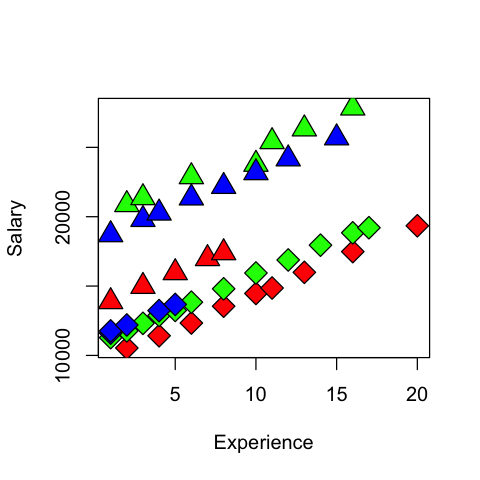## Effect of experience¶ In these pictures, the slope of each line seems to be about the same. How might we estimate it? ### One solution is stratification.¶ • Make six separate models (one for each combination of E and M) and estimate the slope. • Combining them: we could average them? • We have few degrees of freedom in each group. ### Or, use qualitative variables¶ • IF it is reasonable to assume that$\sigma^2$is constant for each observation. • THEN, we can incorporate all observations into 1 model. $$S_i = \beta_0 + \beta_1 X_i + \beta_2 E_{i2} + \beta_3 E_{i3} + \beta_4 M_i + \varepsilon_i$$ Above, the variables are: • $$E_{i2} = \begin{cases} 1 & \text{if E_i=2} \\ 0 & \text{otherwise.} \end{cases}$$ • $$E_{i3} = \begin{cases} 1 & \text{if E_i=3} \\ 0 & \text{otherwise.} \end{cases}$$ • Although$E$has 3 levels, we only added 2 variables to the model. In a sense, this is because (Intercept) (i.e.$\beta_0$) absorbs one level. • If we added three variables then the columns of design matrix would be linearly dependent so we would not have a unique least squares solution. • Assumes$\beta_1$– effect of experience is the same in all groups, unlike when we fit the model separately. This may or may not be reasonable. In : salary.lm <- lm(S ~ E + M + X, salary.table) summary(salary.lm)  Call: lm(formula = S ~ E + M + X, data = salary.table) Residuals: Min 1Q Median 3Q Max -1884.60 -653.60 22.23 844.85 1716.47 Coefficients: Estimate Std. Error t value Pr(>|t|) (Intercept) 8035.60 386.69 20.781 < 2e-16 *** E2 3144.04 361.97 8.686 7.73e-11 *** E3 2996.21 411.75 7.277 6.72e-09 *** M1 6883.53 313.92 21.928 < 2e-16 *** X 546.18 30.52 17.896 < 2e-16 *** --- Signif. codes: 0 ‘***’ 0.001 ‘**’ 0.01 ‘*’ 0.05 ‘.’ 0.1 ‘ ’ 1 Residual standard error: 1027 on 41 degrees of freedom Multiple R-squared: 0.9568, Adjusted R-squared: 0.9525 F-statistic: 226.8 on 4 and 41 DF, p-value: < 2.2e-16  Now, let's take a look at our design matrix In : head(model.matrix(salary.lm))  (Intercept)E2E3M1X 110011 210101 310111 411001 510101 611012 Comparing to our actual data, we can understand how the columns above were formed. They were formed just as we had defined them above. In : head(model.frame(salary.lm))  SEMX 138761 1 1 116083 0 1 187013 1 1 112832 0 1 117673 0 1 208722 1 2 ### Effect of experience¶ • Our model has enforced the constraint the$\beta_1is the same within each group. • Graphically, this seems OK, but how can we test this? • We could fit a model with different slopes in each group, but keeping as many degrees of freedom as we can. • This model has interactions in it: the effect of experience depends on what level of education you have. ### Interaction between experience and education¶ • Model: \begin{aligned} S_i &= \beta_0 + \beta_1 X_i + \beta_2 E_{i2} + \beta_3 E_{i3} +\ \beta_4 M_i \\ & \qquad + \beta_5 E_{i2} X_i + \beta_6 E_{i3} X_i + \varepsilon_i. \end{aligned} • What is the regression function within each group? • Note that we took each column corresponding to education and multiplied it by the column for experience to get two new predictors. • To test whether the slope is the same in each group we would just testH_0:\beta_5 = \beta_6=0$. • Based on figure, we expect not to reject$H_0$. In : model_XE = lm(S~ E + M + X + X:E, salary.table) summary(model_XE)  Call: lm(formula = S ~ E + M + X + X:E, data = salary.table) Residuals: Min 1Q Median 3Q Max -2013.04 -634.68 -16.71 615.66 2014.14 Coefficients: Estimate Std. Error t value Pr(>|t|) (Intercept) 7256.28 549.49 13.205 5.65e-16 *** E2 4172.50 674.97 6.182 2.90e-07 *** E3 3946.36 686.69 5.747 1.16e-06 *** M1 7102.45 333.44 21.300 < 2e-16 *** X 632.29 53.19 11.888 1.53e-14 *** E2:X -125.51 69.86 -1.797 0.0801 . E3:X -141.27 89.28 -1.582 0.1216 --- Signif. codes: 0 ‘***’ 0.001 ‘**’ 0.01 ‘*’ 0.05 ‘.’ 0.1 ‘ ’ 1 Residual standard error: 1005 on 39 degrees of freedom Multiple R-squared: 0.9606, Adjusted R-squared: 0.9546 F-statistic: 158.6 on 6 and 39 DF, p-value: < 2.2e-16  In : anova(salary.lm, model_XE)  Res.DfRSSDfSum of SqFPr(>F) 41 43280719 NA NA NA NA 39 39410680 2 3870040 1.914856 0.1609642 The notation X:E denotes an interaction. Generally, R will take the columns added for E and the columns added for X and add their elementwise product (Hadamard product) to the design matr.x Let's look at our design matrix again to be sure we understand what model was fit. In : model.matrix(model_XE)[10:20,]  (Intercept)E2E3M1XE2:XE3:X 101100330 111001300 121101330 131011303 141000400 151011404 161010404 171100440 181100550 191010505 201001500 ## Remember, it's still a model (i.e. a plane)¶### Interaction between management and education¶ • We can also test for interactions between qualitative variables. • In our plot, note that Master's in management make more than PhD's in management, but this difference disappears in non-management. • This means the effect of education is different in the two management levels. This is evidence of an interaction. • To see this, we plot the residuals within groups separately. In : plot(salary.table$X, salary.table$S, type='n', xlab='Experience', ylab='Salary') colors <- c('red', 'green', 'blue') symbols <- c(23,24) for (i in 1:3) { for (j in 0:1) { subset <- as.logical((salary.table$E == i) * (salary.table$M == j)) points(salary.table$X[subset], salary.table$S[subset], pch=symbols[j+1], bg=colors[i], cex=2) } }In : r = resid(salary.lm) k = 1 plot(salary.table$X, r, xlim=c(1,6), type='n', xlab='Group', ylab='Residuals')
for (i in 1:3) {
for (j in 0:1) {
subset <- as.logical((salary.table$E == i) * (salary.table$M == j))
points(rep(k, length(r[subset])), r[subset], pch=symbols[j+1], bg=colors[i], cex=2)
k = k+1
}
}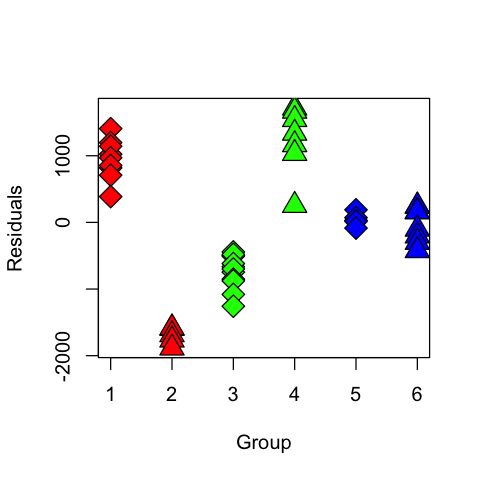R has a special plot that can help visualize this effect, called an interaction.plot.

In :
interaction.plot(salary.table$E, salary.table$M, r, type='b', col=c('red',
'blue'), lwd=2, pch=c(23,24))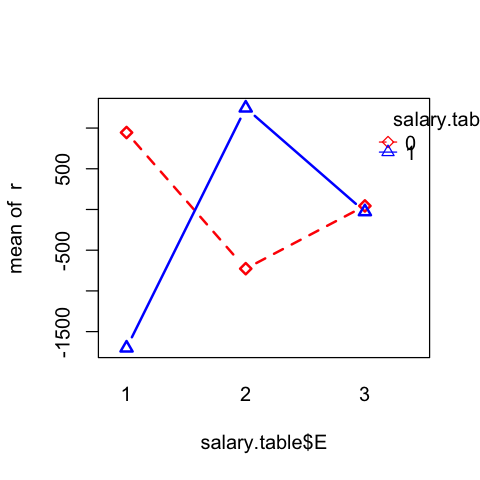### Interaction between management and education¶

• Based on figure, we expect an interaction effect.

• Fit model \begin{aligned} S_i &= \beta_0 + \beta_1 X_i + \beta_2 E_{i2} + \beta_3 E_{i3} +\ \beta_4 M_i \\ & \qquad + \beta_5 E_{i2} M_i + \beta_6 E_{i3} M_i + \varepsilon_i. \end{aligned}

• Again, testing for interaction is testing $H_0:\beta_5=\beta_6=0.$

• What is the regression function within each group?

In :
model_EM = lm(S ~ X + E:M + E + M, salary.table)
summary(model_EM)

Call:
lm(formula = S ~ X + E:M + E + M, data = salary.table)

Residuals:
Min      1Q  Median      3Q     Max
-928.13  -46.21   24.33   65.88  204.89

Coefficients:
Estimate Std. Error t value Pr(>|t|)
(Intercept) 9472.685     80.344  117.90   <2e-16 ***
X            496.987      5.566   89.28   <2e-16 ***
E2          1381.671     77.319   17.87   <2e-16 ***
E3          1730.748    105.334   16.43   <2e-16 ***
M1          3981.377    101.175   39.35   <2e-16 ***
E2:M1       4902.523    131.359   37.32   <2e-16 ***
E3:M1       3066.035    149.330   20.53   <2e-16 ***
---
Signif. codes:  0 ‘***’ 0.001 ‘**’ 0.01 ‘*’ 0.05 ‘.’ 0.1 ‘ ’ 1

Residual standard error: 173.8 on 39 degrees of freedom
Multiple R-squared:  0.9988,	Adjusted R-squared:  0.9986
F-statistic:  5517 on 6 and 39 DF,  p-value: < 2.2e-16

In :
anova(salary.lm, model_EM)

41 43280719 NA NA NA NA
39 1178168 2 42102552 696.8445 3.025504e-31

Let's look at our design matrix again to be sure we understand what model was fit.

In :
head(model.matrix(model_EM))

(Intercept)XE2E3M1E2:M1E3:M1
11100100
21101000
31101101
41110000
51101000
61210110

We will plot the residuals as functions of experience with each experience and management having a different symbol/color.

In :
r = rstandard(model_EM)
plot(salary.table$X, r, type='n') for (i in 1:3) { for (j in 0:1) { subset <- as.logical((salary.table$E == i) * (salary.table$M == j)) points(salary.table$X[subset], r[subset], pch=symbols[j+1], bg=colors[i], cex=2)
}
}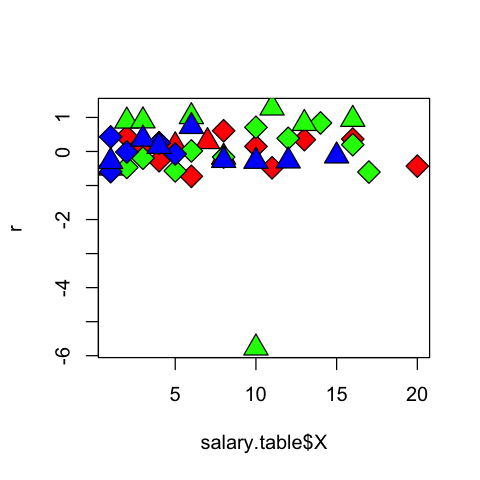One observation seems to be an outlier.

In :
library(car)
outlierTest(model_EM)

    rstudent unadjusted p-value Bonferonni p
33 -14.95083         1.6769e-17    7.714e-16

Let's refit our model to see that our conclusions are not vastly different.

In :
subs33 = c(1:length(salary.table$S))[-33] salary.lm33 = lm(S ~ E + X + M, data=salary.table, subset=subs33) model_EM33 = lm(S ~ E + X + E:M + M, data=salary.table, subset=subs33) anova(salary.lm33, model_EM33)  Res.DfRSSDfSum of SqFPr(>F) 40 43209096.5 NA NA NA NA 38 171188.1 2 43037908 4776.735 2.291239e-46 Let's replot the residuals In : r = rstandard(model_EM33) mf = model.frame(model_EM33) plot(mf$X, r, type='n')
for (i in 1:3) {
for (j in 0:1) {
subset <- as.logical((mf$E == i) * (mf$M == j))
points(mf$X[subset], r[subset], pch=symbols[j+1], bg=colors[i], cex=2) } }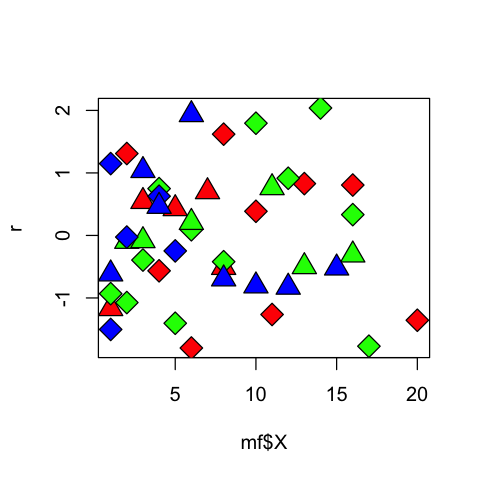Let's make a final plot of the fitted values. In : salaryfinal.lm = lm(S ~ X + E * M, salary.table, subset=subs33) mf = model.frame(salaryfinal.lm) plot(mf$X, mf$S, type='n', xlab='Experience', ylab='Salary') colors <- c('red', 'green', 'blue') ltys <- c(2,3) symbols <- c(23,24) for (i in 1:3) { for (j in 0:1) { subset <- as.logical((mf$E == i) * (mf$M == j)) points(mf$X[subset], mf$S[subset], pch=symbols[j+1], bg=colors[i], cex=2) lines(mf$X[subset], fitted(salaryfinal.lm)[subset], lwd=2, lty=ltys[j], col=colors[i])
}
}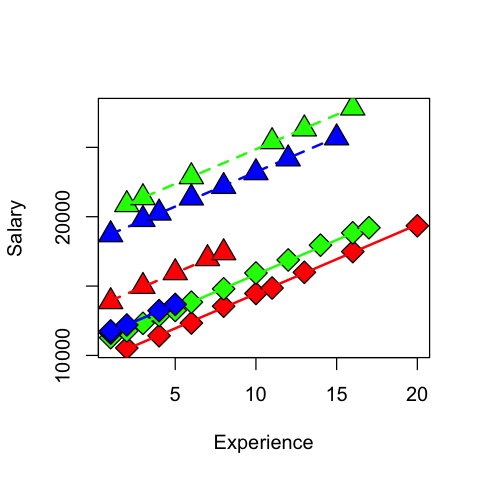### Visualizing an interaction¶

From our first look at the data, the difference between Master's and PhD in the management group is different than in the non-management group. This is an interaction between the two qualitative variables management,M and education,E. We can visualize this by first removing the effect of experience, then plotting the means within each of the 6 groups using interaction.plot.

In :
U = salary.table$S - salary.table$X * model_EM$coef['X'] interaction.plot(salary.table$E, salary.table$M, U, type='b', col=c('red', 'blue'), lwd=2, pch=c(23,24))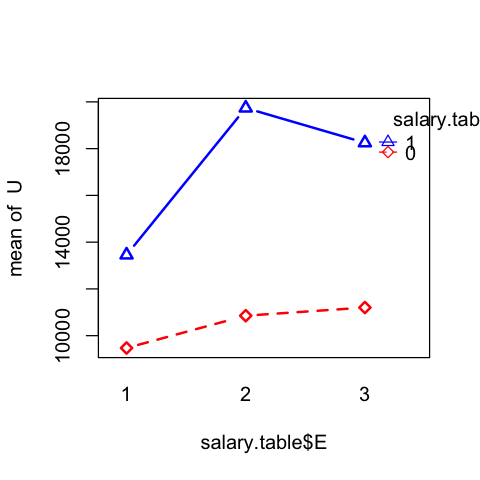### Jobtest employment data (RABE)¶  Variable Description TEST Job aptitude test score MINORITY 1 if applicant could be considered minority, 0 otherwise PERF Job performance evaluation In : url = 'http://stats191.stanford.edu/data/jobtest.table' jobtest.table <- read.table(url, header=T) jobtest.table$MINORITY <- factor(jobtest.table$MINORITY)  Since I will be making several plots, it will be easiest to attach jobtest.table though I will detach it later. These plots would be easier with ggplot. In : attach(jobtest.table) plot(TEST, JPERF, type='n') points(TEST[(MINORITY == 0)], JPERF[(MINORITY == 0)], pch=21, cex=2, bg='purple') points(TEST[(MINORITY == 1)], JPERF[(MINORITY == 1)], pch=25, cex=2, bg='green')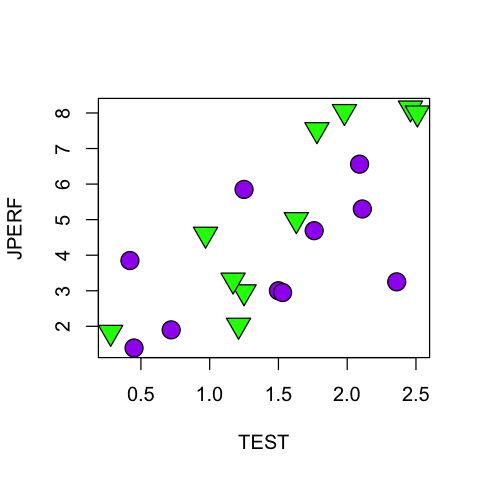### General model¶ • In theory, there may be a linear relationship between$JPERF$and$TEST$but it could be different by group. • Model: $$JPERF_i = \beta_0 + \beta_1 TEST_i + \beta_2 MINORITY_i + \beta_3 MINORITY_i * TEST_i + \varepsilon_i.$$ • Regression functions: $$Y_i = \begin{cases} \beta_0 + \beta_1 TEST_i + \varepsilon_i & \text{if MINORITY_i=0} \\ (\beta_0 + \beta_2) + (\beta_1 + \beta_3) TEST_i + \varepsilon_i & \text{if MINORITY_i=1.} \\ \end{cases}$$ ### Our first model: ($\beta_2=\beta_3=0$)¶ This has no effect for MINORITY. In : jobtest.lm1 <- lm(JPERF ~ TEST, jobtest.table) print(summary(jobtest.lm1))  Call: lm(formula = JPERF ~ TEST, data = jobtest.table) Residuals: Min 1Q Median 3Q Max -3.3558 -0.8798 -0.1897 1.2735 2.3312 Coefficients: Estimate Std. Error t value Pr(>|t|) (Intercept) 1.0350 0.8680 1.192 0.248617 TEST 2.3605 0.5381 4.387 0.000356 *** --- Signif. codes: 0 ‘***’ 0.001 ‘**’ 0.01 ‘*’ 0.05 ‘.’ 0.1 ‘ ’ 1 Residual standard error: 1.591 on 18 degrees of freedom Multiple R-squared: 0.5167, Adjusted R-squared: 0.4899 F-statistic: 19.25 on 1 and 18 DF, p-value: 0.0003555  In : plot(TEST, JPERF, type='n') points(TEST[(MINORITY == 0)], JPERF[(MINORITY == 0)], pch=21, cex=2, bg='purple') points(TEST[(MINORITY == 1)], JPERF[(MINORITY == 1)], pch=25, cex=2, bg='green') abline(jobtest.lm1$coef, lwd=3, col='blue')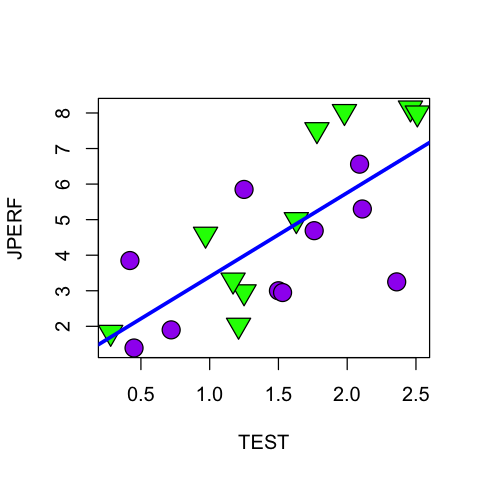### Our second model ($\beta_3=0$)¶

This model allows for an effect of MINORITY but no interaction between MINORITY and TEST.

In :
jobtest.lm2 = lm(JPERF ~ TEST + MINORITY)
print(summary(jobtest.lm2))

Call:
lm(formula = JPERF ~ TEST + MINORITY)

Residuals:
Min      1Q  Median      3Q     Max
-2.7872 -1.0370 -0.2095  0.9198  2.3645

Coefficients:
Estimate Std. Error t value Pr(>|t|)
(Intercept)   0.6120     0.8870   0.690 0.499578
TEST          2.2988     0.5225   4.400 0.000391 ***
MINORITY1     1.0276     0.6909   1.487 0.155246
---
Signif. codes:  0 ‘***’ 0.001 ‘**’ 0.01 ‘*’ 0.05 ‘.’ 0.1 ‘ ’ 1

Residual standard error: 1.54 on 17 degrees of freedom
Multiple R-squared:  0.5724,	Adjusted R-squared:  0.5221
F-statistic: 11.38 on 2 and 17 DF,  p-value: 0.0007312


In :
plot(TEST, JPERF, type='n')
points(TEST[(MINORITY == 0)], JPERF[(MINORITY == 0)], pch=21, cex=2, bg='purple')
points(TEST[(MINORITY == 1)], JPERF[(MINORITY == 1)], pch=25, cex=2, bg='green')
abline(jobtest.lm2$coef['(Intercept)'], jobtest.lm2$coef['TEST'], lwd=3, col='purple')
abline(jobtest.lm2$coef['(Intercept)'] + jobtest.lm2$coef['MINORITY1'], jobtest.lm2$coef['TEST'], lwd=3, col='green')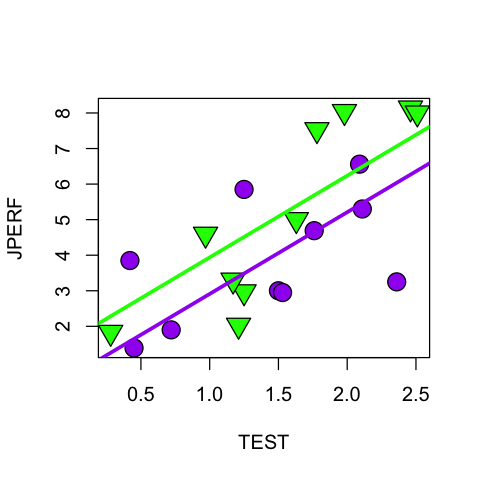### Our third model$(\beta_2=0)$:¶ This model includes an interaction between TEST and MINORITY. These lines have the same intercept but possibly different slopes within the MINORITY groups. In : jobtest.lm3 = lm(JPERF ~ TEST + TEST:MINORITY, jobtest.table) summary(jobtest.lm3)  Call: lm(formula = JPERF ~ TEST + TEST:MINORITY, data = jobtest.table) Residuals: Min 1Q Median 3Q Max -2.41100 -0.88871 -0.03359 0.97720 2.44440 Coefficients: Estimate Std. Error t value Pr(>|t|) (Intercept) 1.1211 0.7804 1.437 0.16900 TEST 1.8276 0.5356 3.412 0.00332 ** TEST:MINORITY1 0.9161 0.3972 2.306 0.03395 * --- Signif. codes: 0 ‘***’ 0.001 ‘**’ 0.01 ‘*’ 0.05 ‘.’ 0.1 ‘ ’ 1 Residual standard error: 1.429 on 17 degrees of freedom Multiple R-squared: 0.6319, Adjusted R-squared: 0.5886 F-statistic: 14.59 on 2 and 17 DF, p-value: 0.0002045  In : plot(TEST, JPERF, type='n') points(TEST[(MINORITY == 0)], JPERF[(MINORITY == 0)], pch=21, cex=2, bg='purple') points(TEST[(MINORITY == 1)], JPERF[(MINORITY == 1)], pch=25, cex=2, bg='green') abline(jobtest.lm3$coef['(Intercept)'], jobtest.lm3$coef['TEST'], lwd=3, col='purple') abline(jobtest.lm3$coef['(Intercept)'], jobtest.lm3$coef['TEST'] + jobtest.lm3$coef['TEST:MINORITY1'], lwd=3, col='green')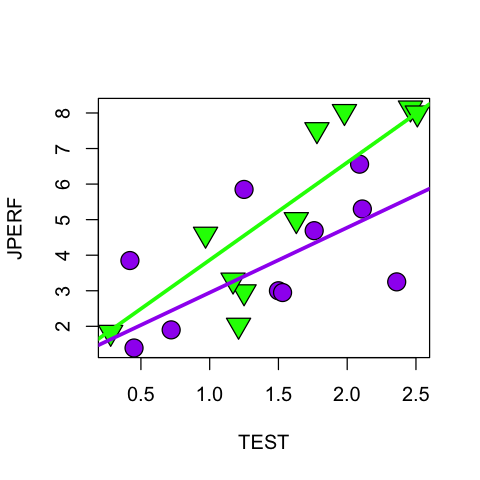Let's look at our design matrix again to be sure we understand which model was fit.

In :
head(model.matrix(jobtest.lm3))

(Intercept)TESTTEST:MINORITY1
11 0.280.28
21 0.970.97
31 1.251.25
41 2.462.46
51 2.512.51
61 1.171.17

### Our final model: no constraints¶

This model allows for different intercepts and different slopes.

In :
jobtest.lm4 = lm(JPERF ~ TEST * MINORITY, jobtest.table)
summary(jobtest.lm4)

Call:
lm(formula = JPERF ~ TEST * MINORITY, data = jobtest.table)

Residuals:
Min      1Q  Median      3Q     Max
-2.0734 -1.0594 -0.2548  1.2830  2.1980

Coefficients:
Estimate Std. Error t value Pr(>|t|)
(Intercept)      2.0103     1.0501   1.914   0.0736 .
TEST             1.3134     0.6704   1.959   0.0677 .
MINORITY1       -1.9132     1.5403  -1.242   0.2321
TEST:MINORITY1   1.9975     0.9544   2.093   0.0527 .
---
Signif. codes:  0 ‘***’ 0.001 ‘**’ 0.01 ‘*’ 0.05 ‘.’ 0.1 ‘ ’ 1

Residual standard error: 1.407 on 16 degrees of freedom
Multiple R-squared:  0.6643,	Adjusted R-squared:  0.6013
F-statistic: 10.55 on 3 and 16 DF,  p-value: 0.0004511

In :
plot(TEST, JPERF, type='n')
points(TEST[(MINORITY == 0)], JPERF[(MINORITY == 0)], pch=21, cex=2, bg='purple')
points(TEST[(MINORITY == 1)], JPERF[(MINORITY == 1)], pch=25, cex=2, bg='green')
abline(jobtest.lm4$coef['(Intercept)'], jobtest.lm4$coef['TEST'], lwd=3, col='purple')
abline(jobtest.lm4$coef['(Intercept)'] + jobtest.lm4$coef['MINORITY1'],
jobtest.lm4$coef['TEST'] + jobtest.lm4$coef['TEST:MINORITY1'], lwd=3, col='green')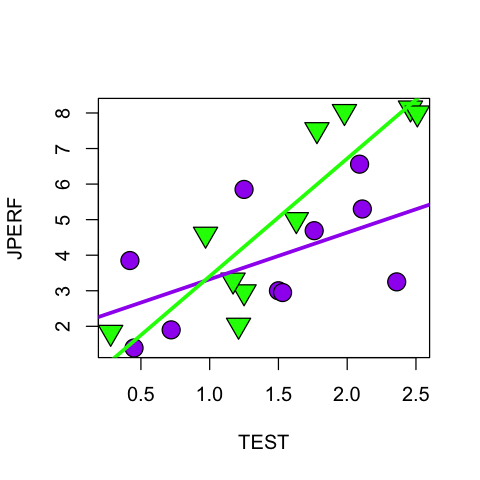The expression TEST*MINORITY is shorthand for TEST + MINORITY + TEST:MINORITY.

In :
head(model.matrix(jobtest.lm4))

(Intercept)TESTMINORITY1TEST:MINORITY1
11 0.281 0.28
21 0.971 0.97
31 1.251 1.25
41 2.461 2.46
51 2.511 2.51
61 1.171 1.17

### Comparing models¶

Is there any effect of MINORITY on slope or intercept?

In :
anova(jobtest.lm1, jobtest.lm4) # ~ TEST to ~ TEST * MINORITY

18 45.56830 NA NA NA NA
16 31.65547 2 13.91282 3.516061 0.05423606

Is there any effect of MINORITY on intercept? (Assuming we have accepted the hypothesis that the slope is the same within each group).

In :
anova(jobtest.lm1, jobtest.lm2) # ~ TEST to ~ TEST + MINORITY

18 45.56830 NA NA NA NA
17 40.32155 1 5.246751 2.212087 0.1552463

We could also have allowed for the possiblity that the slope is different within each group and still check for a different intercept.

In :
anova(jobtest.lm3, jobtest.lm4) # ~ TEST + TEST:MINORITY to
# ~ TEST * MINORITY

17 34.70765 NA NA NA NA
16 31.65547 1 3.05218 1.542699 0.2321149

Is there any effect of MINORITY on slope? (Assuming we have accepted the hypothesis that the intercept is the same within each group).

In :
anova(jobtest.lm1, jobtest.lm3) # ~ TEST vs. ~ TEST + TEST:MINORITY

18 45.56830 NA NA NA NA
17 34.70765 1 10.86064 5.319603 0.03394851

Again, we could have allowed for the possibility that the intercept is different within each group.

In :
anova(jobtest.lm2, jobtest.lm4) # ~ TEST + MINORITY vs.
# ~ TEST * MINORITY

17 40.32155 NA NA NA NA
16 31.65547 1 8.666073 4.380196 0.05265012

In summary, without taking the several tests into account here, there does seem to be some evidence that the slope is different within the two groups.

In :
detach(jobtest.table)


## Model selection¶

Already with this simple dataset (simpler than the IT salary data) we have 4 competing models. How are we going to arrive at a final model?

This highlights the need for model selection. We will come to this topic shortly.